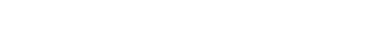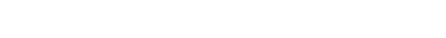Volume 1
1.13. NEUTRALIZATION REACTION. SALTS

Neutralization Reaction. Salts • Partial Neutralization • Exercises
1.13.1. Neutralization Reaction. Salts. Acids readily react with bases. The reaction between an acid and a base represents one of the most important transformations in chemistry. In this reaction, an H atom on an acid remainder and an OH group of a metal hydroxide come together to form a molecule of water. The other product of the reaction is a salt, a chemical compound comprising the acid remainder and the metal atom bonded to each other (Figure 1-48).Figure 1-48. General scheme of the reaction between a base and an acid.

A typical simple acid-base neutralization is the reaction between hydrochloric acid, HCl, and sodium hydroxide, NaOH (Figure 1-49). In this reaction, a highly corrosive acid (HCl) reacts with a highly caustic base (NaOH) to give water and NaCl, a salt. We say that the resultant solution is neutral because it is neither acidic nor basic. That is why the reaction between an acid and a base is called neutralization.Figure 1-49. Neutralization reaction between NaOH (base) and HCl (acid).

Most acids and salts are soluble in water, whereas most bases are not. If an insoluble base reacts with a soluble acid to give a soluble salt (which is frequently the case), the base dissolves as the reaction occurs. As an example, copper hydroxide, Cu(OH)2, a water-insoluble base, dissolves upon treatment with HCl, H2SO4, or HNO3 owing to the formation of the corresponding soluble salt (Figure 1-50).Figure 1-50. Neutralization of Cu(OH)2 with HCl, H2SO4, and HNO3.

Did you notice that while the valence of copper can be 1 or 2, the Cu(OH)2 above was named just copper hydroxide, not copper (II) hydroxide? The valence of the copper was not included in the name because copper (I) hydroxide (CuOH) does not exist! When saying "copper hydroxide", we do not have to specify the valence of the copper because it can only be Cu(OH)2.

Most salts are soluble in water, but some are not. Examples of water-insoluble salts include silver chloride (AgCl), calcium carbonate (CaCO3), and barium sulfate (BaSO4).

1.13.2. Partial Neutralization. What would happen if a dibasic acid was treated with a monobasic base in a 1 to 1 ratio? In such a case, the amount of the base is enough to neutralize only one of the two hydrogen atoms on the acid molecule (Figure 1-51).Figure 1-51. Partial neutralization of a dibasic acid with a monobasic base.

A typical example of such "partial neutralization" is presented in Figure 1-52. The product of this reaction, NaHSO4, is a hydro salt. The full chemical name for this salt is sodium hydrogen sulfate or sodium hydrosulfate or sodium bisulfate. Another example of a hydro salt is baking soda, NaHCO3, sodium hydrogen carbonate or sodium hydrocarbonate or sodium bicarbonate.Figure 1-52. Partial neutralization of H2SO4 with NaOH.

Similarly, if there is not enough acid to neutralize all of the OH groups on a metal hydroxide, a hydroxo salt is formed. The beautiful green mineral malachite mentioned above is a naturally occurring hydroxo salt, Cu2(OH)2CO3.

1.13.3. Exercises.

1. Balance the following chemical equations for neutralization reactions between an acid and a base.

(a) Mg(OH)2 + HCl = MgCl2 + H2O

(b) KOH + H3PO4 = K3PO4 + H2O

(c) Ca(OH)2 + HNO3 = Ca(NO3)2 + H2O

(d) Ba(OH)2 + H2SO4 = BaSO4↓ + H2O

(e) Al(OH)3 + H2SO4 = Al2(SO4)3 + H2O

2. How much HBr is needed to neutralize 60 g of NaOH? Answer

3. 50 g of a 7% solution of HCl was mixed with 30 g of a 9% solution of KOH. A piece of blue litmus paper was then dipped in the resultant solution. Did the litmus paper color change to red? Answer